# 十、使用 OpenCV 的机器学习

• 数据量大大增加。
• 有明显改进的算法。
• 实质上有更强大的计算机硬件。 虚拟个人助理（例如，智能扬声器或移动应用），通勤时的预测（交通预测或导航服务），视频系统（监控摄像头系统或车牌识别系统）以及电子商务应用（推荐系统或自动价格识别系统） 比较应用）只是我们日常生活中机器学习应用的一些示例。

• 机器学习入门
• K 均值聚类
• K 最近邻
• 支持向量机

# 技术要求

• Python 和 OpenCV
• 特定于 Python 的 IDE
• NumPy 和 Matplotlib 包
• Git 客户端

# 机器学习入门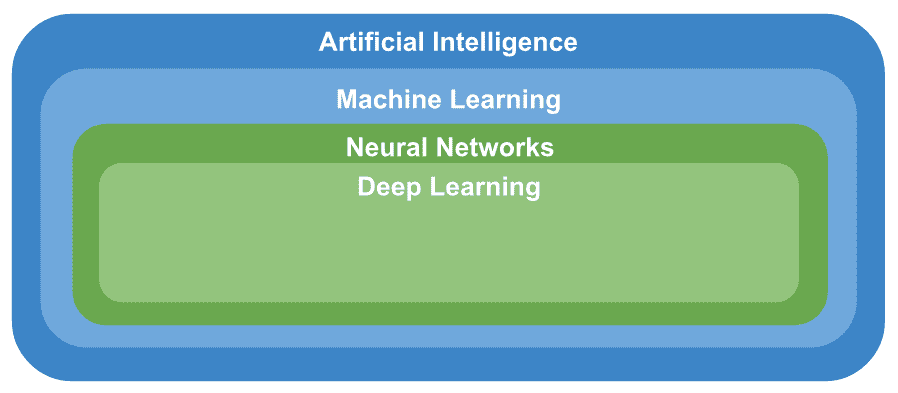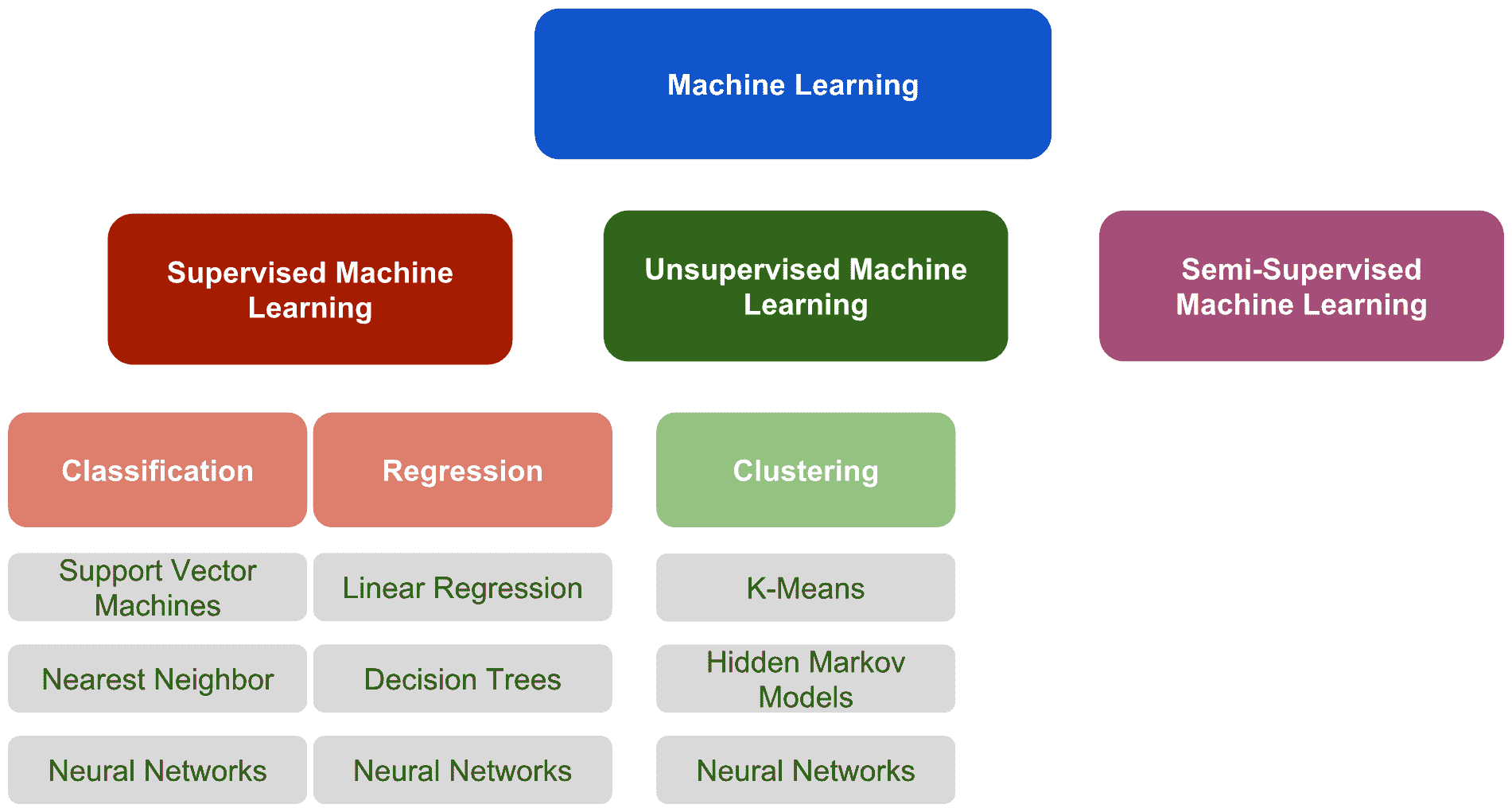# 监督机器学习

• 分类：当输出变量是类别（例如颜色（红色，绿色或蓝色），尺寸（大，中或小）或性别（男性或女性））时，可能被视为分类问题。 在分类问题中，该算法将输入映射到输出标签。
• 回归：当输出变量是真实值（例如年龄或体重）时，监督学习问题可以归类为回归问题。 在回归问题中，该算法将输入映射到连续输出。

• 偏差方差权衡：偏差方差折衷是机器学习中的一个常用术语，指的是模型-数据不足的模型具有较高的偏差，而模型的数据过拟合则偏高。 数据差异很大：
• 偏差可以看作是学习算法中错误假设产生的误差，可以定义为模型预测与我们尝试预测的正确值之间的差异。 这导致算法通过不考虑数据中的所有信息（拟合不足）来学习错误的东西。 因此，具有高偏差的模型无法在数据中找到所有模式，因此它不太适合训练集，也不太适合测试集。
• 方差可以定义为算法通过拟合模型来密切学习数据中的误差/噪声（过拟合），而该趋势倾向于学习错误的事物，而与真实信号无关。 因此，具有高方差的模型非常适合训练集，但是由于它也已获悉数据中的误差/噪声，因此无法推广到测试集。 请查看下图，以更好地理解：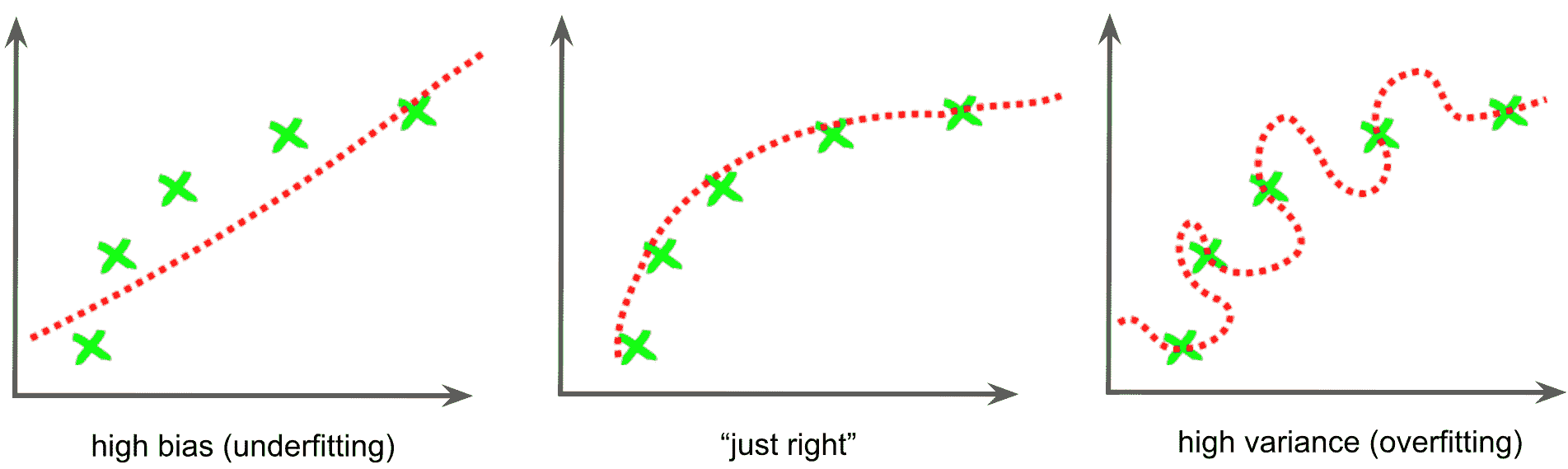• 函数复杂度和训练数据量模型复杂度是指机器学习算法正尝试以与多项式次数相似的方式学习函数的复杂性。 模型复杂性的适当级别通常由训练数据的性质决定。 例如，如果您需要少量的数据来训练模型，则低复杂度的模型是可取的。 这是因为高复杂度模型将适合较小的训练集。
• 输入空间的维数：在处理高/非常高维的特征空间时，学习问题可能会非常困难，因为许多额外的特征会混淆学习过程， 结果差异很大。 因此，当处理高/非常高维的特征空间时，一种常见的方法是将学习算法修改为具有高偏差和低方差。 此问题与维度诅咒有关，后者指的是在分析和组织在低维空间中找不到的高维空间中的数据时出现的各个方面。
• 输出值中的噪声：如果所需的输出值不正确（由于人为或传感器误差），则学习算法尝试过于紧密地拟合数据时，可能会发生过拟合。 有几种常见的策略可用于减轻输出值中的误差/噪声影响。 例如，在训练算法之前检测并去除嘈杂的训练示例是一种常见的方法。 另一个策略是尽早停止，可以用来防止过拟合。

# 半监督机器学习

• 您可以使用无监督学习技术来发现和学习输入变量中的结构。
• 您可以使用监督学习技术来使用标记的数据训练分类器，然后使用此模型对未标记的数据进行预测。 此时，您可以将该数据作为训练数据反馈到监督学习算法中，以迭代地增加标记数据的大小，并使用重新训练的模型对新的未标记数据进行预测。

# K 均值聚类

OpenCV 提供cv2.kmeans()函数，该函数实现了 K 均值聚类算法，该算法查找聚类的中心并对聚类周围的输入样本进行分组。

K 均值聚类算法的目标是将n个样本划分（或聚类）为K聚类，其中每个样本将属于具有最均值的聚类。 cv2.kmeans()函数的签名如下：

retval, bestLabels, centers=cv.kmeans(data, K, bestLabels, criteria, attempts, flags[, centers])


data代表用于聚类的输入数据。 它应为np.float32数据类型，并且每个特征都应放在单个列中。 K指定最后所需的群集数。 使用criteria参数指定算法终止标准，该参数设置最大迭代次数和/或所需的精度。 当满足这些条件时，算法终止。 criteria是三个参数typemax_itermepsilon的元组：

• type：这是终止条件的类型。 它具有三个标志：
• cv2.TERM_CRITERIA_EPS：当达到指定的精度epsilon时，算法停止。
• cv2.TERM_CRITERIA_MAX_ITER：当达到指定的迭代次数max_iterm时，算法停止。
• cv2.TERM_CRITERIA_EPS + cv2.TERM_CRITERIA_MAX_ITER：达到两个条件中的任何一个时，算法将停止。
• max_iterm：这是最大迭代次数。
• epsilon：这是必需的精度。

criteria = (cv2.TERM_CRITERIA_EPS + cv2.TERM_CRITERIA_MAX_ITER, 20, 1.0)


attempts参数指定使用不同的初始标签执行算法的次数。 该算法返回产生最佳紧密度的标签。 flags参数指定如何获取初始中心。 cv2.KMEANS_RANDOM_CENTERS标志在每次尝试中选择随机的初始中心。 cv2.KMEANS_PP_CENTERS标志使用 Arthur 和 Vassilvitskii 提出的 K 均值++ 中心初始化（请参阅《K 均值++：精心播种的优势》（2007））。

cv2.kmeans()返回以下内容：

• bestLabels：一个整数数组，存储每个样本的聚类索引
• centers：一个数组，其中包含每个集群的中心
• compactness：每个点到其相应中心的距离的平方之和

# 了解 K 均值聚类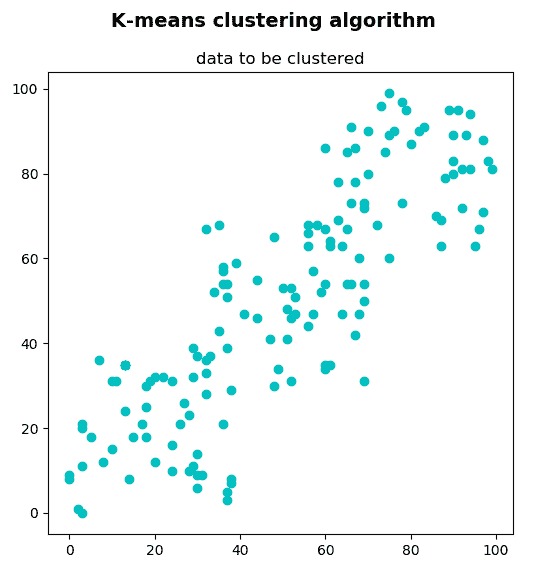data = np.float32(np.vstack(
(np.random.randint(0, 40, (50, 2)), np.random.randint(30, 70, (50, 2)), np.random.randint(60, 100, (50, 2)))))


• k_means_clustering_k_2.py：在此脚本中，data已分为两个组（K = 2）。
• k_means_clustering_k_3.py：在此脚本中，data已分为三个组（K = 3）。
• k_means_clustering_k_4.py：在此脚本中，data已分为四个组（K = 4）。

k_means_clustering_k_2.py脚本中，数据已集群为2集群。 第一步是定义算法终止标准。 在这种情况下，最大迭代次数设置为20max_iterm = 20），ε设置为1.0epsilon = 1.0）：

criteria = (cv2.TERM_CRITERIA_EPS + cv2.TERM_CRITERIA_MAX_ITER, 20, 1.0)


ret, label, center = cv2.kmeans(data, 2, None, criteria, 10, cv2.KMEANS_RANDOM_CENTERS)


A = data[label.ravel() == 0]
B = data[label.ravel() == 1]


# Create the dimensions of the figure and set title:
fig = plt.figure(figsize=(12, 6))
plt.suptitle("K-means clustering algorithm", fontsize=14, fontweight='bold')
fig.patch.set_facecolor('silver')

# Plot the 'original' data:
ax = plt.subplot(1, 2, 1)
plt.scatter(data[:, 0], data[:, 1], c='c')
plt.title("data")

# Plot the 'clustered' data and the centroids
ax = plt.subplot(1, 2, 2)
plt.scatter(A[:, 0], A[:, 1], c='b')
plt.scatter(B[:, 0], B[:, 1], c='g')
plt.scatter(center[:, 0], center[:, 1], s=100, c='m', marker='s')
plt.title("clustered data and centroids (K = 2)")

# Show the Figure:
plt.show()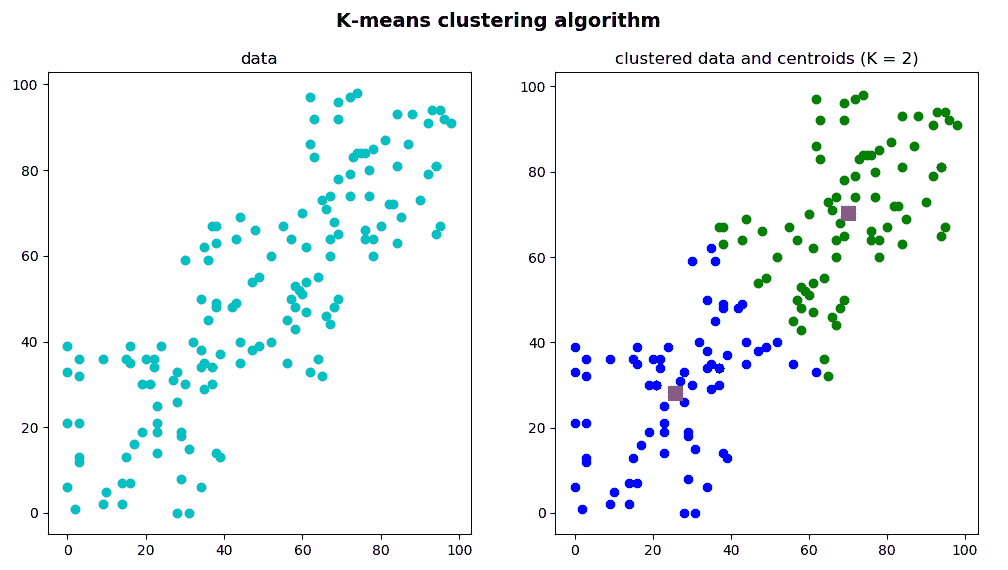k_means_clustering_k_3.py脚本中，采用了相同的步骤对数据进行聚类，但是我们决定将数据分组为3聚类（K = 3）。 因此，在调用cv2.kmeans()函数时，K参数设置为3

ret, label, center = cv2.kmeans(data, 3, None, criteria, 10, cv2.KMEANS_RANDOM_CENTERS)


A = data[label.ravel() == 0]
B = data[label.ravel() == 1]
C = data[label.ravel() == 2]


# Create the dimensions of the figure and set title:
fig = plt.figure(figsize=(12, 6))
plt.suptitle("K-means clustering algorithm", fontsize=14, fontweight='bold')
fig.patch.set_facecolor('silver')

# Plot the 'original' data:
ax = plt.subplot(1, 2, 1)
plt.scatter(data[:, 0], data[:, 1], c='c')
plt.title("data")

# Plot the 'clustered' data and the centroids
ax = plt.subplot(1, 2, 2)
plt.scatter(A[:, 0], A[:, 1], c='b')
plt.scatter(B[:, 0], B[:, 1], c='g')
plt.scatter(C[:, 0], C[:, 1], c='r')
plt.scatter(center[:, 0], center[:, 1], s=100, c='m', marker='s')
plt.title("clustered data and centroids (K = 3)")

# Show the Figure:
plt.show()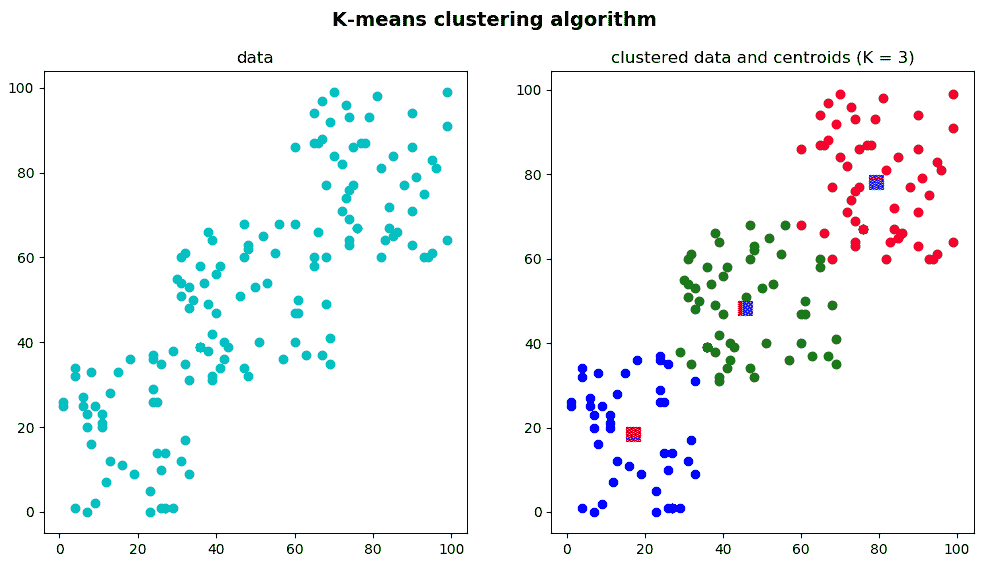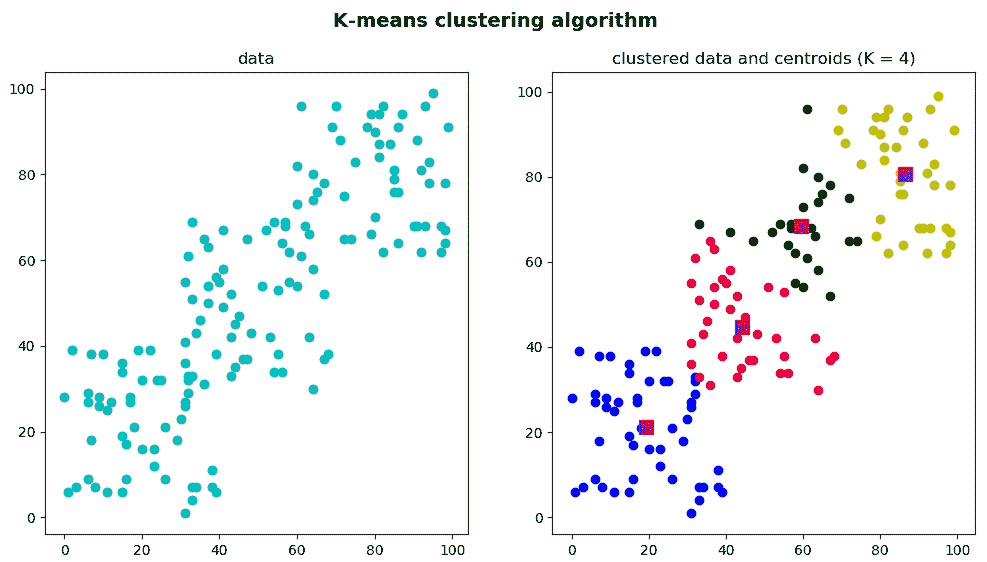# 使用 K 均值聚类的颜色量化

k_means_color_quantization.py脚本中，我们执行 K 均值聚类算法以执行颜色量化。 在这种情况下，数据的每个元素都由3特征组成，这些特征对应于图像每个像素的BGR值。 因此，关键步骤是通过以下方式将图像转换为data

data = np.float32(image).reshape((-1, 3))


1. 加载 BGR 图片：
img = cv2.imread('landscape_1.jpg')

1. 使用color_quantization()函数执行色彩量化：
color_3 = color_quantization(img, 3)

1. 同时显示两个图像以查看结果。 color_quantization()函数执行颜色量化过程：
def color_quantization(image, k):
"""Performs color quantization using K-means clustering algorithm"""

# Transform image into 'data':
data = np.float32(image).reshape((-1, 3))
# print(data.shape)

# Define the algorithm termination criteria (maximum number of iterations and/or required accuracy):
# In this case the maximum number of iterations is set to 20 and epsilon = 1.0
criteria = (cv2.TERM_CRITERIA_EPS + cv2.TERM_CRITERIA_MAX_ITER, 20, 1.0)

# Apply K-means clustering algorithm:
ret, label, center = cv2.kmeans(data, k, None, criteria, 10, cv2.KMEANS_RANDOM_CENTERS)

# At this point we can make the image with k colors
# Convert center to uint8:
center = np.uint8(center)
# Replace pixel values with their center value:
result = center[label.flatten()]
result = result.reshape(img.shape)
return result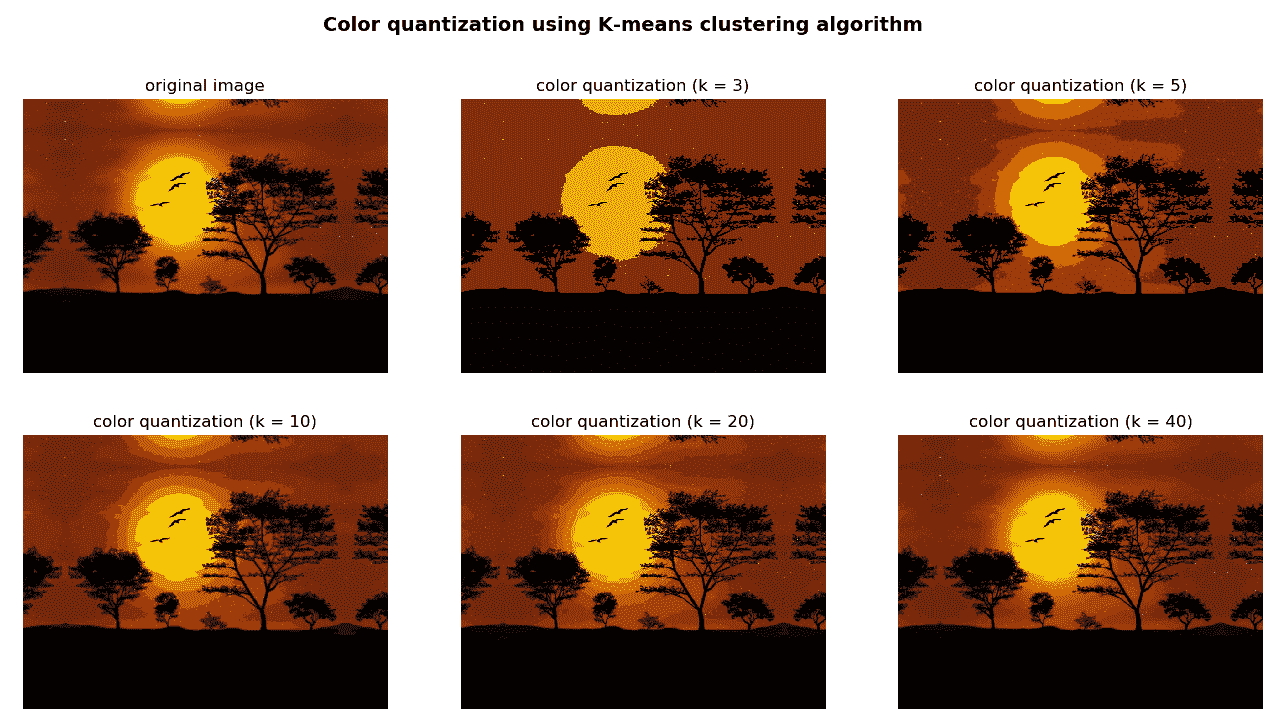color_quantization()函数已被修改为包括以下功能：

def color_quantization(image, k):
"""Performs color quantization using K-means clustering algorithm"""

# Transform image into 'data':
data = np.float32(image).reshape((-1, 3))
# print(data.shape)

# Define the algorithm termination criteria (the maximum number of iterations and/or the desired accuracy):
# In this case the maximum number of iterations is set to 20 and epsilon = 1.0
criteria = (cv2.TERM_CRITERIA_EPS + cv2.TERM_CRITERIA_MAX_ITER, 20, 1.0)

# Apply K-means clustering algorithm:
ret, label, center = cv2.kmeans(data, k, None, criteria, 10, cv2.KMEANS_RANDOM_CENTERS)

# At this point we can make the image with k colors
# Convert center to uint8:
center = np.uint8(center)
# Replace pixel values with their center value:
result = center[label.flatten()]
result = result.reshape(img.shape)

# Build the 'color_distribution' legend.
# We will use the number of pixels assigned to each center value:
counter = collections.Counter(label.flatten())
print(counter)

# Calculate the total number of pixels of the input image:
total = img.shape * img.shape

# Assign width and height to the color_distribution image:
desired_width = img.shape
# The difference between 'desired_height' and 'desired_height_colors'
# will be the separation between the images
desired_height = 70
desired_height_colors = 50

# Initialize the color_distribution image:
color_distribution = np.ones((desired_height, desired_width, 3), dtype="uint8") * 255
# Initialize start:
start = 0

for key, value in counter.items():
# Calculate the normalized value:
value_normalized = value / total * desired_width

# Move end to the right position:
end = start + value_normalized

# Draw rectangle corresponding to the current color:
cv2.rectangle(color_distribution, (int(start), 0), (int(end), desired_height_colors), center[key].tolist(), -1)
# Update start:
start = end

return np.vstack((color_distribution, result))


counter = collections.Counter(label.flatten())


np.vstack((color_distribution, result))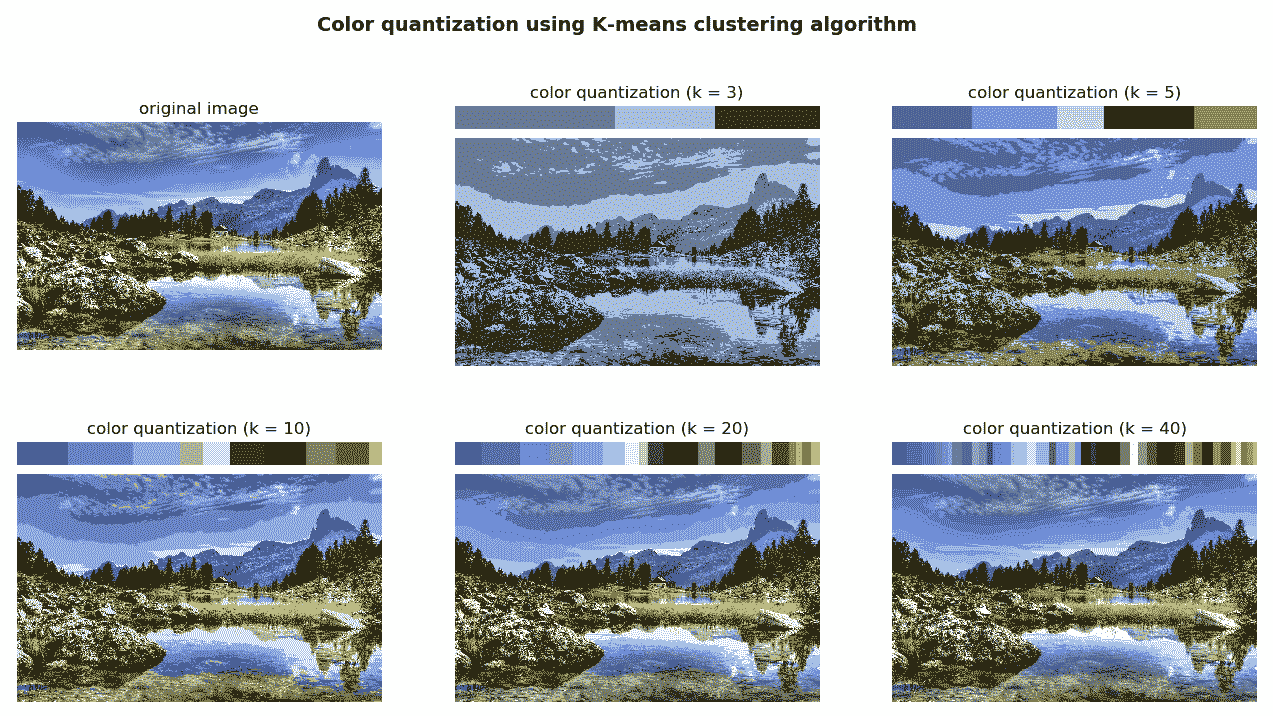# K 最近邻

K 最近邻kNN）被认为是有监督学习类别中最简单的算法之一。 kNN 可用于分类和回归问题。 在训练阶段，kNN 同时存储所有训练样本的特征向量和类别标签。 在分类阶段，将未标记向量（与训练示例位于同一多维特征空间中的查询或测试向量）分类为最接近要分类的未标记向量的k个训练样本中最频繁的类别标签，其中k是用户定义的常数。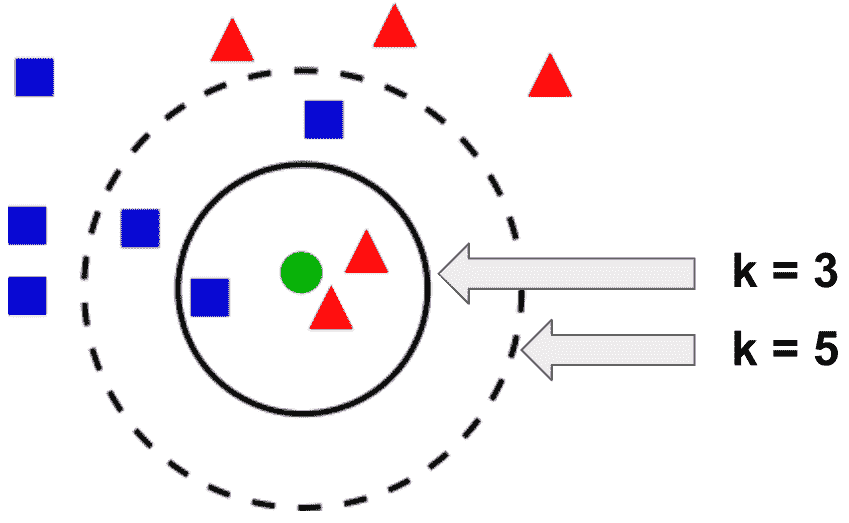retval, results, neighborResponses, dist=cv2.ml_KNearest.findNearest(samples, k[, results[, neighborResponses[, dist]]])


# 了解 K 最近邻

knn_introduction.py脚本对 kNN 进行了简单介绍，其中随机创建了一组点并分配了一个标签（01）。 标签0将代表红色三角形，而标签1将代表蓝色正方形。 我们将使用 kNN 算法基于k最近邻对样本点进行分类。

# The data is composed of 16 points:
data = np.random.randint(0, 100, (16, 2)).astype(np.float32)

# We create the labels (0: red, 1: blue) for each of the 16 points:
labels = np.random.randint(0, 2, (16, 1)).astype(np.float32)

# Create the sample point to be classified:
sample = np.random.randint(0, 100, (1, 2)).astype(np.float32)


# KNN creation:
knn = cv2.ml.KNearest_create()
# KNN training:
knn.train(data, cv2.ml.ROW_SAMPLE, labels)
# KNN find nearest:
k = 3
ret, results, neighbours, dist = knn.findNearest(sample, k)

# Print results:
print("result: {}".format(results))
print("neighbours: {}".format(neighbours))
print("distance: {}".format(dist))


result: [[0.]]
neighbours: [[0\. 0\. 0.]]
distance: [[ 80\. 100\. 196.]]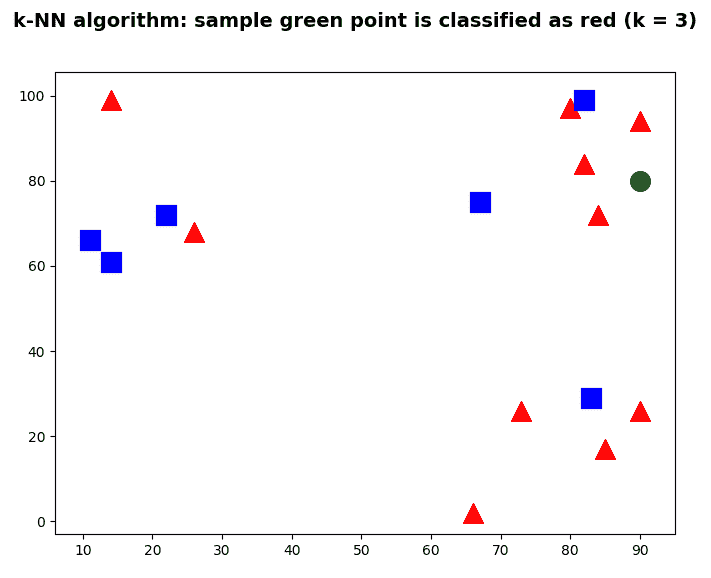# 使用 K 最近邻识别手写数字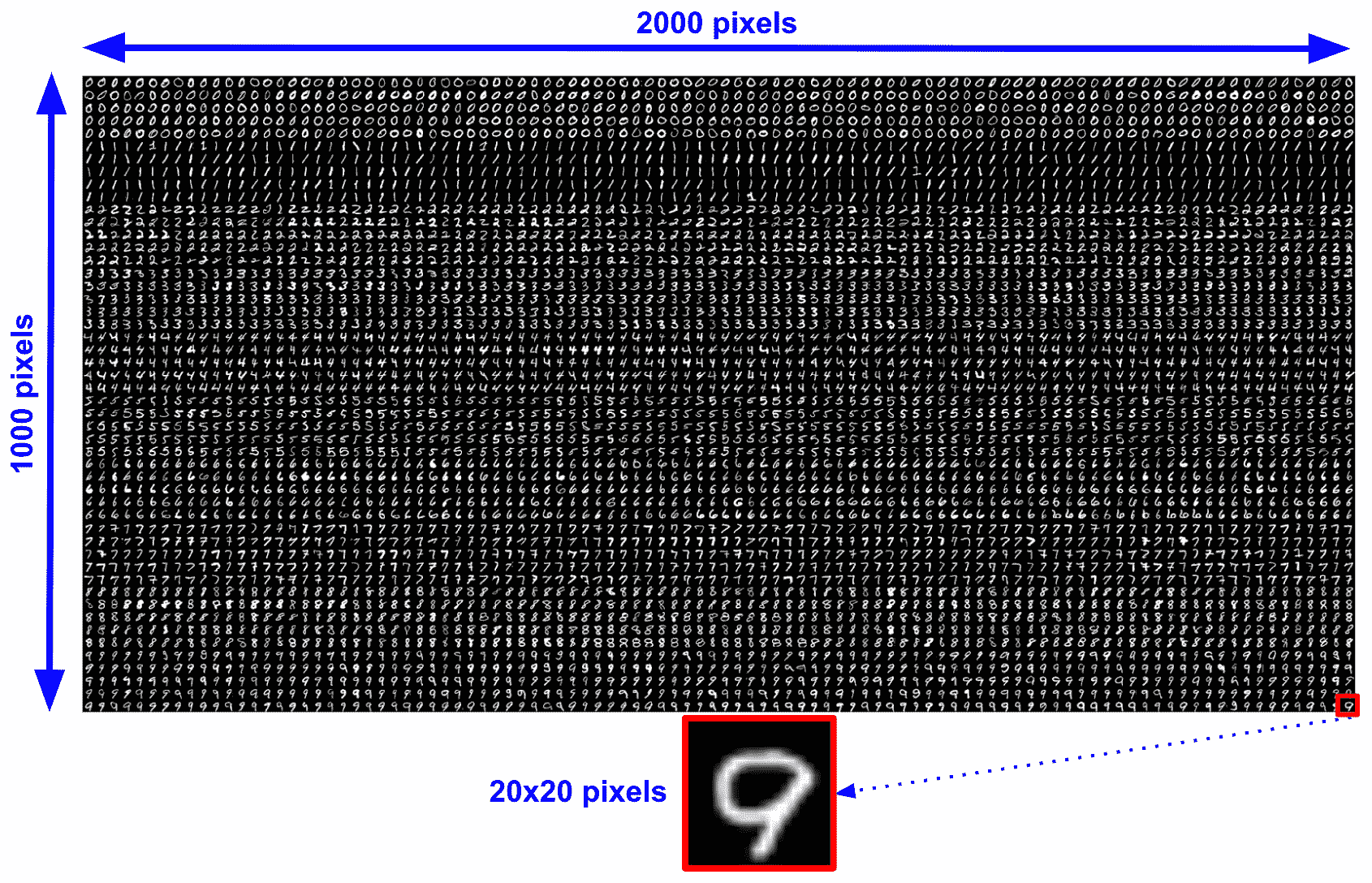knn_handwritten_digits_recognition_introduction.py脚本中，我们将执行第一种方法，尝试使用 kNN 分类器识别数字。 在第一种方法中，我们将使用原始像素值作为特征。 这样，每个描述符的大小将为 400（20 x 20）。

digits, labels = load_digits_and_labels('digits.png')


load_digits_and_labels()函数的代码如下：

def load_digits_and_labels(big_image):
"""Returns all the digits from the 'big' image and creates the corresponding labels for each image"""

# Load the 'big' image containing all the digits:

# Get all the digit images from the 'big' image:
number_rows = digits_img.shape / SIZE_IMAGE
rows = np.vsplit(digits_img, digits_img.shape / SIZE_IMAGE)

digits = []
for row in rows:
row_cells = np.hsplit(row, number_rows)
for digit in row_cells:
digits.append(digit)
digits = np.array(digits)

# Create the labels for each image:
labels = np.repeat(np.arange(NUMBER_CLASSES), len(digits) / NUMBER_CLASSES)
return digits, labels


# Compute the descriptors for all the images.
# In this case, the raw pixels are the feature descriptors
raw_descriptors = []
for img in digits:
raw_descriptors.append(np.float32(raw_pixels(img)))
raw_descriptors = np.squeeze(raw_descriptors)


partition = int(0.5 * len(raw_descriptors))
raw_descriptors_train, raw_descriptors_test = np.split(raw_descriptors, [partition])
labels_train, labels_test = np.split(labels, [partition])


# Train the KNN model:
print('Training KNN model - raw pixels as features')
knn = cv2.ml.KNearest_create()
knn.train(raw_descriptors_train, cv2.ml.ROW_SAMPLE, labels_train)

# Test the created model:
k = 5
ret, result, neighbours, dist = knn.findNearest(raw_descriptors_test, k)

# Compute the accuracy:
acc = get_accuracy(result, labels_test)
print("Accuracy: {}".format(acc))


results = defaultdict(list)


from collections import defaultdict


for k in np.arange(1, 10):
ret, result, neighbours, dist = knn.findNearest(raw_descriptors_test, k)
acc = get_accuracy(result, labels_test)
print(" {}".format("%.2f" % acc))
results['50'].append(acc)


# Show all results using matplotlib capabilities:
fig, ax = plt.subplots(1, 1)
ax.set_xlim(0, 10)
dim = np.arange(1, 10)

for key in results:
ax.plot(dim, results[key], linestyle='--', marker='o', label="50%")

plt.legend(loc='upper left', title="% training")
plt.title('Accuracy of the KNN model varying k')
plt.xlabel("number of k")
plt.ylabel("accuracy")
plt.show()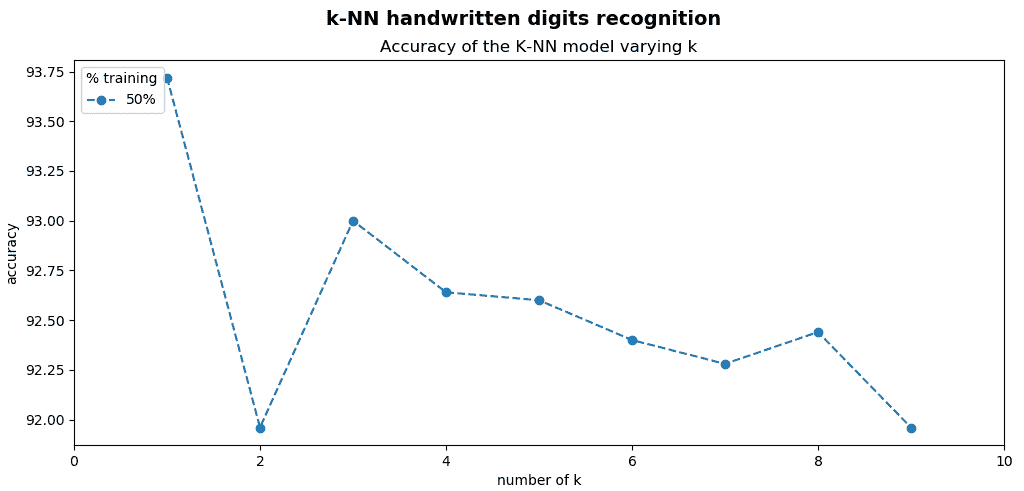knn_handwritten_digits_recognition_k_training_testing.py脚本中，我们修改了图像的百分比以训练和测试模型，如下所示：

# Split data into training/testing:
split_values = np.arange(0.1, 1, 0.1)

for split_value in split_values:
# Split the data into training and testing:
partition = int(split_value * len(raw_descriptors))
raw_descriptors_train, raw_descriptors_test = np.split(raw_descriptors, [partition])
labels_train, labels_test = np.split(labels, [partition])

# Train KNN model
print('Training KNN model - raw pixels as features')
knn.train(raw_descriptors_train, cv2.ml.ROW_SAMPLE, labels_train)

# Store the accuracy when testing:
for k in np.arange(1, 10):
ret, result, neighbours, dist = knn.findNearest(raw_descriptors_test, k)
acc = get_accuracy(result, labels_test)
print(" {}".format("%.2f" % acc))
results[int(split_value * 100)].append(acc)


# Show all results using matplotlib capabilities:
# Create the dimensions of the figure and set title:
fig = plt.figure(figsize=(12, 5))
plt.suptitle("KNN handwritten digits recognition", fontsize=14, fontweight='bold')
fig.patch.set_facecolor('silver')

ax = plt.subplot(1, 1, 1)
ax.set_xlim(0, 10)
dim = np.arange(1, 10)

for key in results:
ax.plot(dim, results[key], linestyle='--', marker='o', label=str(key) + "%")

plt.legend(loc='upper left', title="% training")
plt.title('Accuracy of the KNN model varying both k and the percentage of images to train/test')
plt.xlabel("number of k")
plt.ylabel("accuracy")
plt.show()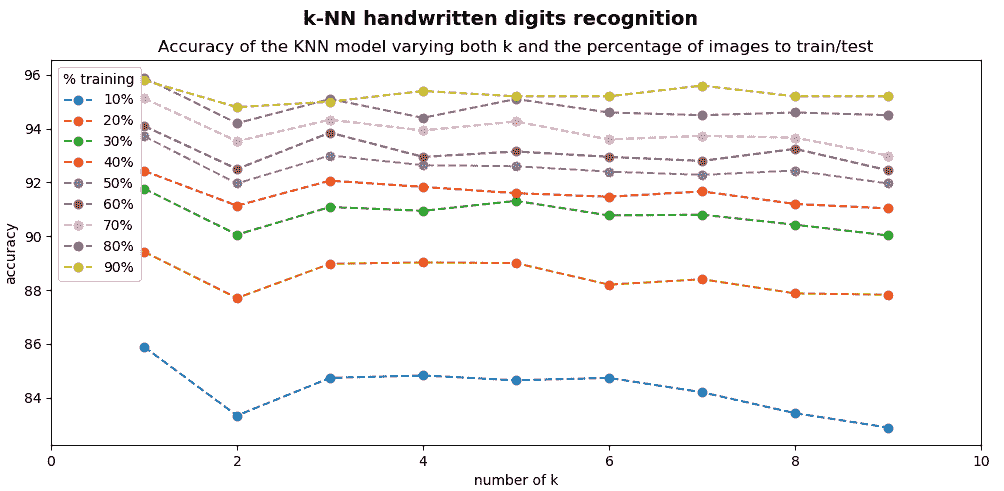def deskew(img):
"""Pre-processing of the images"""

m = cv2.moments(img)
if abs(m['mu02']) < 1e-2:
return img.copy()
skew = m['mu11'] / m['mu02']
M = np.float32([[1, skew, -0.5 * SIZE_IMAGE * skew], [0, 1, 0]])
img = cv2.warpAffine(img, M, (SIZE_IMAGE, SIZE_IMAGE), flags=cv2.WARP_INVERSE_MAP | cv2.INTER_LINEAR)
return img


deskew()函数通过使用二阶矩来使数字偏斜。 更具体地，可以通过两个中心矩之比（mu11/mu02）来计算偏斜的量度。 计算出的偏斜度用于计算仿射变换，从而使数字偏斜。 请参阅下一个屏幕截图，以欣赏此预处理的效果。 屏幕截图的顶部显示了原始数字（蓝色边框），屏幕截图的底部显示了预处理的数字（绿色边框）：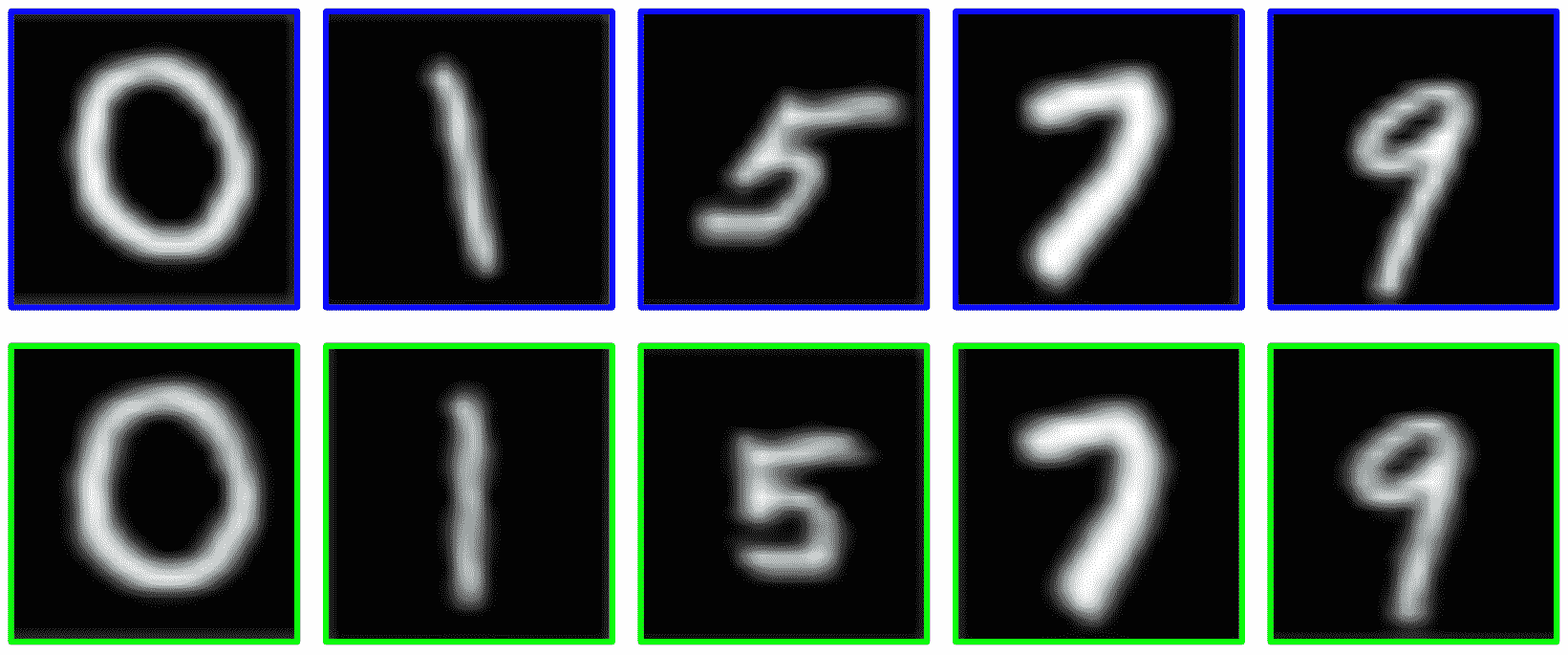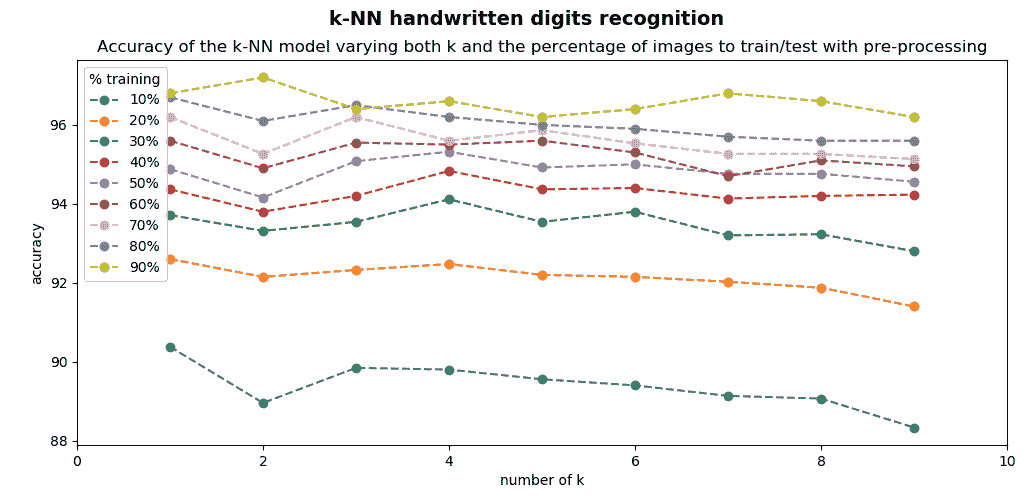HOG 是一种用于计算机视觉的流行特征描述符，最早用于人类在静态图像中的检测。 在knn_handwritten_digits_recognition_k_training_testing_preprocessing_hog.py脚本中，我们将使用 HOG 特征代替原始像素值。

def get_hog():
"""Get hog descriptor"""

# cv2.HOGDescriptor(winSize, blockSize, blockStride, cellSize, nbins, derivAperture, winSigma, histogramNormType,
hog = cv2.HOGDescriptor((SIZE_IMAGE, SIZE_IMAGE), (8, 8), (4, 4), (8, 8), 9, 1, -1, 0, 0.2, 1, 64, True)
print("hog descriptor size: '{}'".format(hog.getDescriptorSize()))
return hog


# Compute the descriptors for all the images.
# In this case, the HoG descriptor is calculated
hog_descriptors = []
for img in digits:
hog_descriptors.append(hog.compute(deskew(img)))
hog_descriptors = np.squeeze(hog_descriptors)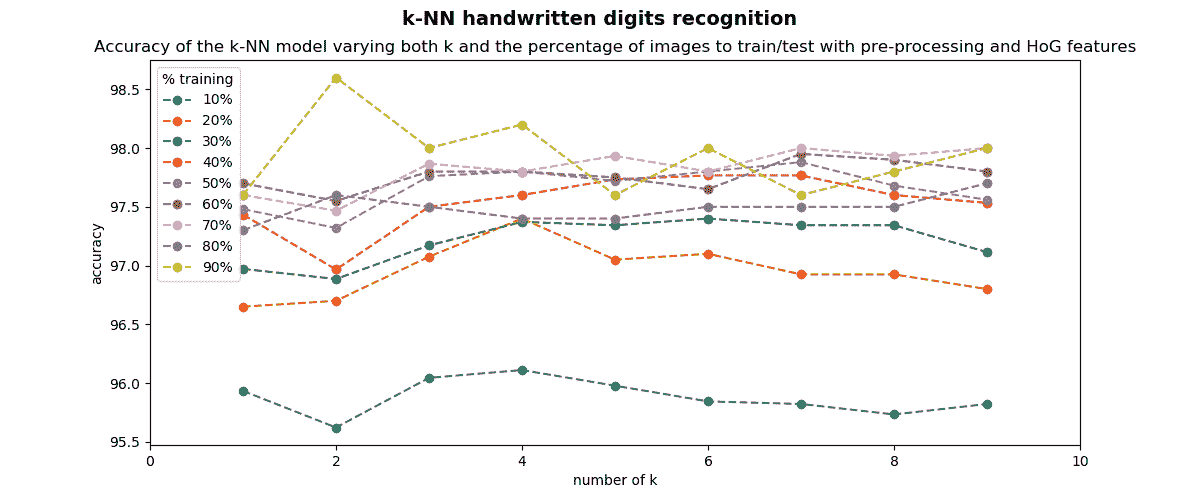k=2和 90% 的数字用于训练，而 10% 的数字用于测试时，则达到 98.60% 的精度。 因此，我们将识别率从 92.60%（在本小节的第一个脚本中获得）提高到 98.60%（在上一个脚本中获得）。

# 支持向量机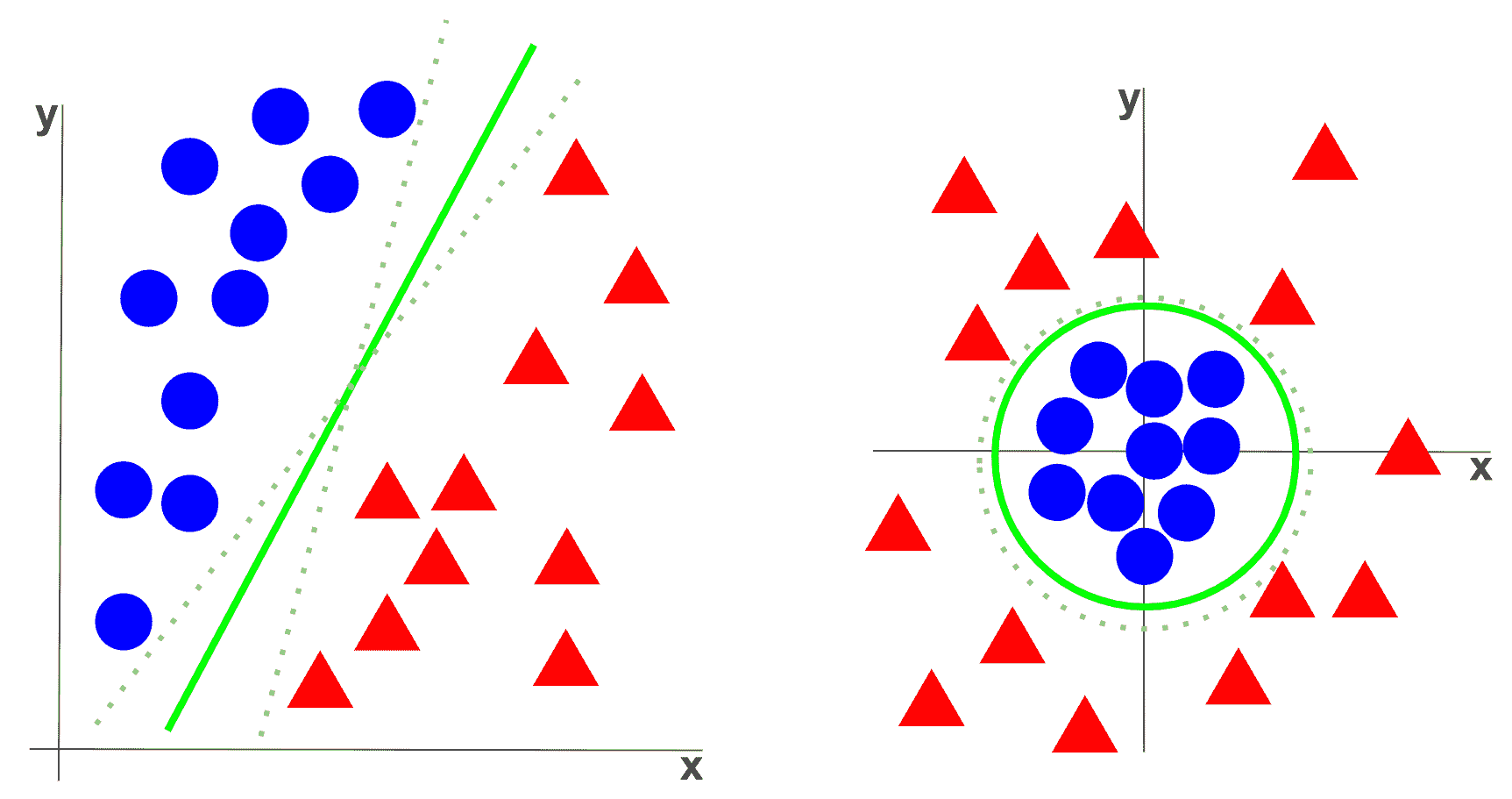OpenCV 中的 SVM 实现基于《LIBSVM：支持向量机库》（2011）。 要创建空模型，请使用cv2.ml.SVM_create()函数。 接下来，应将主要参数分配给模型：

• svmType：这设置 SVM 的类型。 有关详细信息，请参见 LibSVM。 可能的值如下：
• SVM_C_SVC：可用于n类分类的 C-支持向量分类（n ≥ 2
• NU_SVC：ν-支持向量分类
• ONE_CLASS：分布估计（一类 SVM）
• EPS_SVR：ε-支持向量回归
• NU_SVR：ν-支持向量回归
• kernelType：设置 SVM 的核类型。 有关详细信息，请参见 LibSVM。 可能的值如下：
• LINEAR：线性核
• POLY：多项式核
• RBF径向基函数RBF），在大多数情况下是个不错的选择
• SIGMOID：Sigmoid 核
• CHI2：指数 Chi2 核，类似于RBF
• INTER：直方图交点核； 快速核

• degree：核函数的参数度（POLY
• gamma：核函数的γ参数（POLY/RBF/SIGMOID/CHI2
• coef0：核函数的coef0参数（POLY/SIGMOID
• Cvalue：SVM 优化问题的C参数（C_SVC/EPS_SVR/NU_SVR

• nu：SVM 优化问题的ν参数（NU_SVC/ONE_CLASS/NU_SVR

• p：SVM 优化问题（EPS_SVR）的ε参数
• classWeightsC_SVC问题中的可选权重，分配给特定类别
• termCrit：SVM 迭代训练过程的终止标准

svmType: C_SVC, kernelType: RBF, degree: 0, gamma: 1, coef0: 0, C: 1, nu: 0, p: 0, classWeights: 0, termCrit: TermCriteria(MAX_ITER+EPS, 1000, FLT_EPSILON )


# 了解 SVM

svm_introduction.py脚本执行一个简单的示例，以了解如何在 OpenCV 中使用 SVM。 首先，我们创建训练数据和标签：

# Set up training data:
labels = np.array([1, 1, -1, -1, -1])
data = np.matrix([[500, 10], [550, 100], [300, 10], [500, 300], [10, 600]], dtype=np.float32)


# Initialize the SVM model:
svm_model = svm_init(C=12.5, gamma=0.50625)


svm_init()函数创建一个空模型并分配主要参数并返回模型：

def svm_init(C=12.5, gamma=0.50625):
"""Creates empty model and assigns main parameters"""

model = cv2.ml.SVM_create()
model.setGamma(gamma)
model.setC(C)
model.setKernel(cv2.ml.SVM_LINEAR)
model.setType(cv2.ml.SVM_C_SVC)
model.setTermCriteria((cv2.TERM_CRITERIA_MAX_ITER, 100, 1e-6))

return model


# Train the SVM:
svm_train(svm_model, data, labels)


def svm_train(model, samples, responses):
"""Trains the model using the samples and the responses"""

model.train(samples, cv2.ml.ROW_SAMPLE, responses)
return model


# Create the canvas (black image with three channels)
# This image will be used to show the prediction for every pixel:
img_output = np.zeros((640, 640, 3), dtype="uint8")


# Show the SVM response:
show_svm_response(svm_model, img_output)


def show_svm_response(model, image):
"""Show the prediction for every pixel of the image, the training data and the support vectors"""

colors = {1: (255, 255, 0), -1: (0, 255, 255)}

# Show the prediction for every pixel of the image:
for i in range(image.shape):
for j in range(image.shape):
sample = np.matrix([[j, i]], dtype=np.float32)
response = svm_predict(model, sample)

image[i, j] = colors[response.item(0)]

# Show the training data:
# Show samples with class 1:
cv2.circle(image, (500, 10), 10, (255, 0, 0), -1)
cv2.circle(image, (550, 100), 10, (255, 0, 0), -1)
# Show samples with class -1:
cv2.circle(image, (300, 10), 10, (0, 255, 0), -1)
cv2.circle(image, (500, 300), 10, (0, 255, 0), -1)
cv2.circle(image, (10, 600), 10, (0, 255, 0), -1)

# Show the support vectors:
support_vectors = model.getUncompressedSupportVectors()
for i in range(support_vectors.shape):
cv2.circle(image, (support_vectors[i, 0], support_vectors[i, 1]), 15, (0, 0, 255), 6)


• 图像每个像素的预测
• 所有五个训练数据点
• 支持向量（定义超平面的向量称为支持向量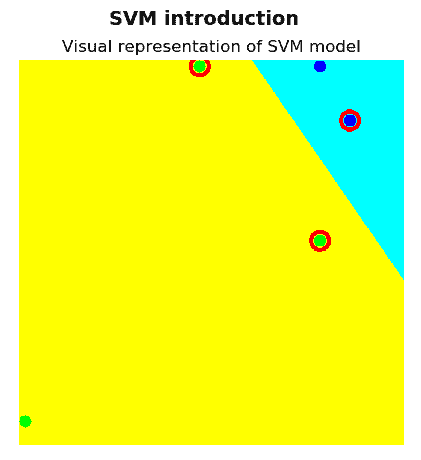# 使用 SVM 的手写数字识别

svm_handwritten_digits_recognition_preprocessing_hog.py脚本使用 SVM 分类执行手写数字识别。 关键代码如下所示：

# Load all the digits and the corresponding labels:

# Shuffle data
# Constructs a random number generator:
rand = np.random.RandomState(1234)
# Randomly permute the sequence:
shuffle = rand.permutation(len(digits))
digits, labels = digits[shuffle], labels[shuffle]

# HoG feature descriptor:
hog = get_hog()

# Compute the descriptors for all the images.
# In this case, the HoG descriptor is calculated
hog_descriptors = []
for img in digits:
hog_descriptors.append(hog.compute(deskew(img)))
hog_descriptors = np.squeeze(hog_descriptors)

# At this point we split the data into training and testing (50% for each one):
partition = int(0.5 * len(hog_descriptors))
hog_descriptors_train, hog_descriptors_test = np.split(hog_descriptors, [partition])
labels_train, labels_test = np.split(labels, [partition])

print('Training SVM model ...')
model = svm_init(C=12.5, gamma=0.50625)
svm_train(model, hog_descriptors_train, labels_train)

print('Evaluating model ... ')
svm_evaluate(model, hog_descriptors_test, labels_test)


def svm_init(C=12.5, gamma=0.50625):
"""Creates empty model and assigns main parameters"""

model = cv2.ml.SVM_create()
model.setGamma(gamma)
model.setC(C)
model.setKernel(cv2.ml.SVM_RBF)
model.setType(cv2.ml.SVM_C_SVC)
model.setTermCriteria((cv2.TERM_CRITERIA_MAX_ITER, 100, 1e-6))

return model


svm_handwritten_digits_recognition_preprocessing_hog.py脚本相比，在svm_handwritten_digits_recognition_preprocessing_hog_c_gamma.py脚本中进行了两次修改。 第一个是使用 90% 的数字训练模型，其余 10% 用于测试。 第二个修改是对Cγ进行网格搜索：

# Create a dictionary to store the accuracy when testing:
results = defaultdict(list)

for C in [1, 10, 100, 1000]:
for gamma in [0.1, 0.3, 0.5, 0.7, 0.9, 1.1, 1.3, 1.5]:
model = svm_init(C, gamma)
svm_train(model, hog_descriptors_train, labels_train)
acc = svm_evaluate(model, hog_descriptors_test, labels_test)
print(" {}".format("%.2f" % acc))
results[C].append(acc)


# Create the dimensions of the figure and set title:
fig = plt.figure(figsize=(10, 6))
plt.suptitle("SVM handwritten digits recognition", fontsize=14, fontweight='bold')
fig.patch.set_facecolor('silver')

# Show all results using matplotlib capabilities:
ax = plt.subplot(1, 1, 1)
ax.set_xlim(0, 1.5)
dim = [0.1, 0.3, 0.5, 0.7, 0.9, 1.1, 1.3, 1.5]

for key in results:
ax.plot(dim, results[key], linestyle='--', marker='o', label=str(key))

plt.legend(loc='upper left', title="C")
plt.title('Accuracy of the SVM model varying both C and gamma')
plt.xlabel("gamma")
plt.ylabel("accuracy")
plt.show()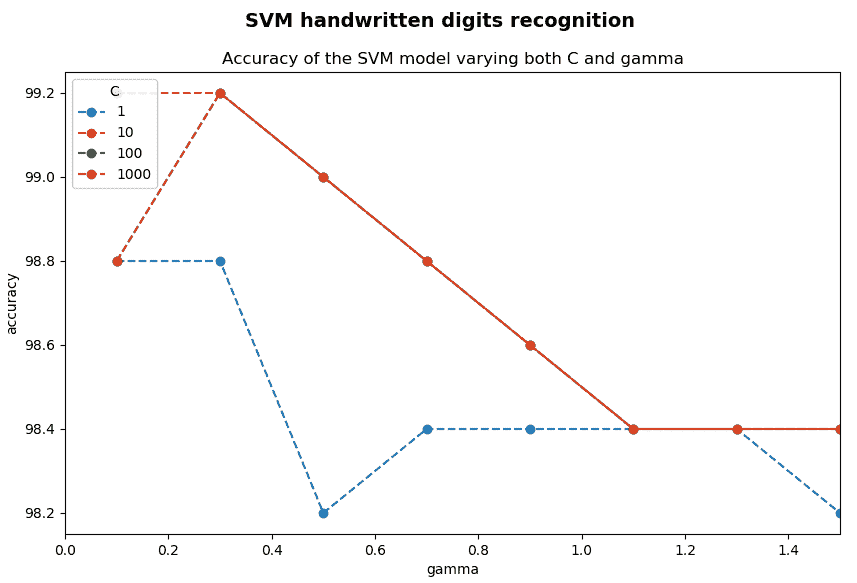# 问题

1. 机器学习中的三种主要方法是什么？
2. 分类和回归问题有什么区别？
3. OpenCV 提供什么函数来实现 K 均值聚类算法？
4. OpenCV 提供什么函数来创建 kNN 分类器？
5. OpenCV 提供什么函数来找到最近的邻居？
6. OpenCV 提供什么函数来创建 SVM 分类器？
7. SVM 核的合理首选是什么？